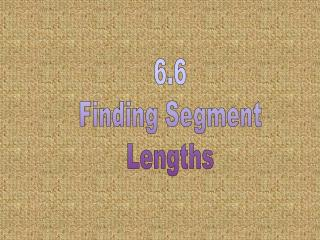DownloadDownload Presentation6.6 Finding Segment Lengths

# 6.6 Finding Segment Lengths

Download Presentation## 6.6 Finding Segment Lengths

- - - - - - - - - - - - - - - - - - - - - - - - - - - E N D - - - - - - - - - - - - - - - - - - - - - - - - - - -
##### Presentation Transcript

1. 6.6 Finding Segment Lengths

2. Chord-Chord Rule: Two chords intersect INSIDE the circle a ab = cd d c b

3. Example 1: 9 12 6 3 x x 2 2 X = 3 X = 8 x 3 6 2 X = 1

4. 12 2x 8 3x Example 2: Find x 2x  3x = 12  8 6x2 = 96 x2 = 16 x = 4

5. Secant-Secant Rule: OW-OW

6. Two secants intersect OUTSIDE the circle Secant-Secant Rule: E A B C D EA•EB = EC•ED

7. Example 3: B 13 A 7 E 4 C x D 7 (7 + 13) = 4 (4 + x) x = 31 140 = 16 + 4x 124 = 4x

8. Example 4: B x A 5 D 8 6 C E 6 (6 + 8) = 5 (5 + x) x = 11.8 84 = 25 + 5x 59 = 5x

9. Notice that on the tangent segment, the outside is the whole! Secant Segment External Segment Tangent Segment

10. Secant-Tangent Rule: A secant and tangent originate from the same point OUTSIDE the circle.

11. A secant and tangent originate from the same point OUTSIDE the circle Secant-Tangent Rule: C B E A EA2= EB • EC

12. Example 5: C B x 12 E 24 A (12 + x) 242 = 12 x = 36 576 = 144 + 12x

13. Example 6: 5 B E 15 C x A (5 + 15) x2 = 5 x = 10 x2 = 100

14. What you should know by now… Given two chords Given two secants ORa tangent and a secant